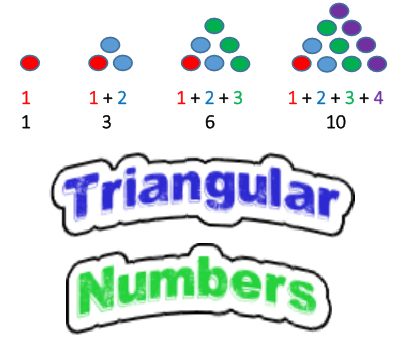Learn about triangular numbers and their extraordinary applications. Discover its relationship with Combinations. and solve some interesting puzzles based on this concept.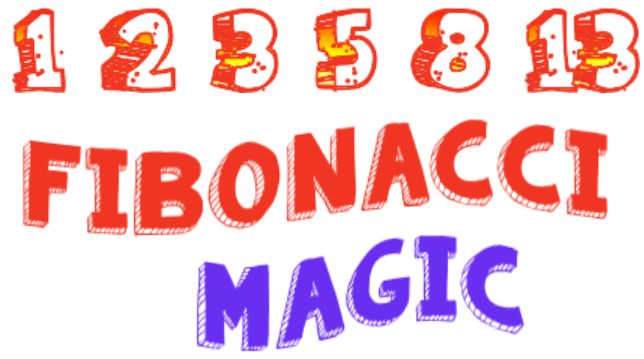Learn one of the characteristics of Fibonacci Numbers and how this characteristic is used in Fibonacci Coding. A simple magic card trick based on the same concept in store at the end.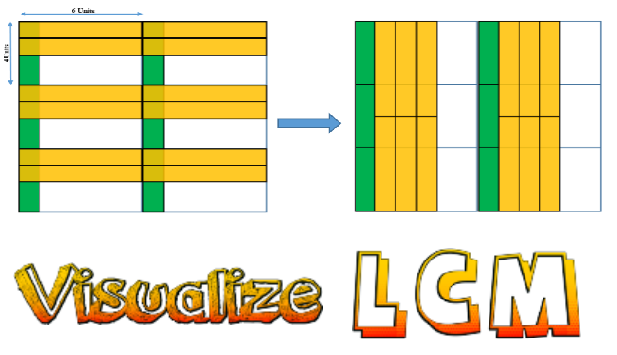Learn the concept of LCM visually, and also understand why LCM needs to be used when solving certain type of problems and puzzles.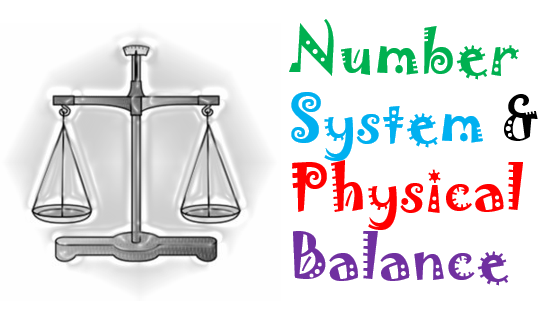Understand the number system concept behind the puzzles related to physical balance and weights.Learn about Progressions and their extra-ordinary use in metric conversions.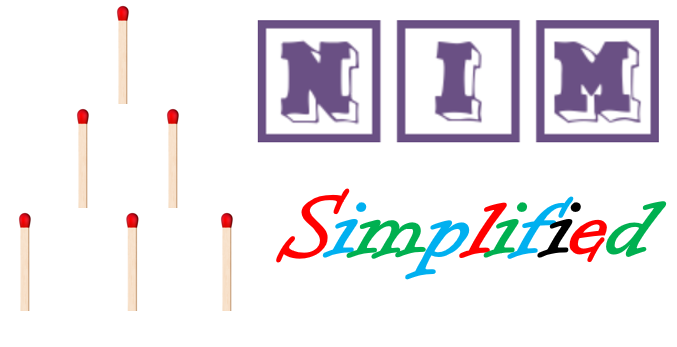A Simplified NIM Game that helps you understand the math behind the classical NIM.